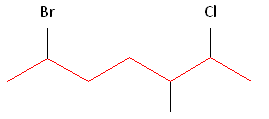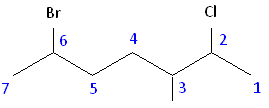2.4: Naming Alkanes

Objectives

After completing this section, you should be able to

1. provide the correct IUPAC name for any given alkane structure (Kekulé, condensed or shorthand).
2. draw the Kekulé, condensed or shorthand structure of an alkane, given its IUPAC name.

Key Terms

Make certain that you can define, and use in context, the key term below.

• IUPAC system

Study Notes

The IUPAC system of nomenclature aims to ensure

1. that every organic compound has a unique, unambiguous name.
2. that the IUPAC name of any compound conveys the structure of that compound to a person familiar with the system.

One way of checking whether the name you have given to an alkane is reasonable is to count the number of carbon atoms implied by the chosen name. For example, if you named a compound 3‑ethyl-4‑methylheptane, you have indicated that the compound contains a total of 10 carbon atoms—seven carbon atoms in the main chain, two carbon atoms in an ethyl group, and one carbon atom in a methyl group. If you were to check the given structure and find 11 carbon atoms, you would know that you had made a mistake. Perhaps the name you should have written was 3‑ethyl-4,4‑dimethylheptane!

When naming alkanes, a common error of beginning students is a failure to pick out the longest carbon chain. For example, the correct name for the compound shown below is 3‑methylheptane, not 2‑ethylhexane.Remember that every substituent must have a number, and do not forget the prefixes: di, tri, tetra, etc.

You must use commas to separate numbers, and hyphens to separate numbers and substituents. Notice that 3‑methylhexane is one word.

Hydrocarbons having no double or triple bond functional groups are classified as alkanes or cycloalkanes, depending on whether the carbon atoms of the molecule are arranged only in chains or also in rings. Although these hydrocarbons have no functional groups, they constitute the framework on which functional groups are located in other classes of compounds, and provide an ideal starting point for studying and naming organic compounds. The alkanes and cycloalkanes are also members of a larger class of compounds referred to as aliphatic. Simply put, aliphatic compounds are compounds that do not incorporate any aromatic rings in their molecular structure.

The following table lists the IUPAC names assigned to simple continuous-chain alkanes from C-1 to C-10. A common "ane" suffix identifies these compounds as alkanes. Longer chain alkanes are well known, and their names may be found in many reference and text books. The names methane through decane should be memorized, since they constitute the root of many IUPAC names. Fortunately, common numerical prefixes are used in naming chains of five or more carbon atoms.

Table $$\PageIndex{1}$$: Simple Unbranched Alkanes
Name Molecular
Formula
Structural
Formula
Isomers   Name Molecular
Formula
Structural
Formula
Isomers
methane CH4 CH4 1   hexane C6H14 CH3(CH2)4CH3 5
ethane C2H6 CH3CH3 1   heptane C7H16 CH3(CH2)5CH3 9
propane C3H8 CH3CH2CH3 1   octane C8H18 CH3(CH2)6CH3 18
butane C4H10 CH3CH2CH2CH3 2   nonane C9H20 CH3(CH2)7CH3 35
pentane C5H12 CH3(CH2)3CH3 3   decane C10H22 CH3(CH2)8CH3 75

Some important behavior trends and terminologies

1. The formulas and structures of these alkanes increase uniformly by a CH2 increment.
2. A uniform variation of this kind in a series of compounds is called homologous.
3. These formulas all fit the CnH2n+2 rule. This is also the highest possible H/C ratio for a stable hydrocarbon.
4. Since the H/C ratio in these compounds is at a maximum, we call them saturated (with hydrogen).

Beginning with butane (C4H10), and becoming more numerous with larger alkanes, we note the existence of alkane isomers. For example, there are five C6H14 isomers, shown below as abbreviated line formulas (A through E):Although these distinct compounds all have the same molecular formula, only one (A) can be called hexane. How then are we to name the others?

The IUPAC system requires first that we have names for simple unbranched chains, as noted above, and second that we have names for simple alkyl groups that may be attached to the chains. Examples of some common alkyl groups are given in the following table. Note that the "ane" suffix is replaced by "yl" in naming groups. The symbol R is used to designate a generic (unspecified) alkyl group.

 Group Name CH3– C2H5– CH3CH2CH2– (CH3)2CH– CH3CH2CH2CH2– (CH3)2CHCH2– CH3CH2CH(CH3)– (CH3)3C– R– Methyl Ethyl Propyl Isopropyl Butyl Isobutyl sec-Butyl tert-Butyl Alkyl

IUPAC Rules for Alkane Nomenclature

1. Find and name the longest continuous carbon chain.
2. Identify and name groups attached to this chain.
3. Number the chain consecutively, starting at the end nearest a substituent group.
4. Designate the location of each substituent group by an appropriate number and name.
5. Assemble the name, listing groups in alphabetical order.
6. The prefixes di, tri, tetra etc., used to designate several groups of the same kind, are not considered when alphabetizing.

Example $$\PageIndex{1}$$: AlkanesThe IUPAC names of the isomers of hexane are: A hexane B 2-methylpentane C 3-methylpentane D 2,2-dimethylbutane E 2,3-dimethylbutane

Alkyl Groups

Halogen substituents are easily accommodated, using the names: fluoro (F-), chloro (Cl-), bromo (Br-) and iodo (I-).

Example $$\PageIndex{2}$$: Halogen Substitution

For example, (CH3)2CHCH2CH2Br would be named 1-bromo-3-methylbutane. If the halogen is bonded to a simple alkyl group an alternative "alkyl halide" name may be used. Thus, C2H5Cl may be named chloroethane (no locator number is needed for a two carbon chain) or ethyl chloride.

Alkyl Groups

Alkanes can be described by the general formula CnH2n+2. An alkyl group is formed by removing one hydrogen from the alkane chain and is described by the formula CnH2n+1. The removal of this hydrogen results in a stem change from -ane to -yl. Take a look at the following examples.The same concept can be applied to any of the straight chain alkane names provided in the table above.

Name Molecular Formula Condensed Structural Formula
Methane CH4 CH4
Ethane C2H6 CH3CH3
Propane C3H8 CH3CH2CH3
Butane C4H10 CH3(CH2)2CH3
Pentane C5H12 CH3(CH2)3CH3
Hexane C6H14 CH3(CH2)4CH3
Heptane C7H16 CH3(CH2)5CH3
Octane C8H18 CH3(CH2)6CH3
Nonane C9H20 CH3(CH2)7CH3
Decane C10H22 CH3(CH2)8CH3
Undecane C11H24 CH3(CH2)9CH3
Dodecane C12H26 CH3(CH2)10CH3
Tridecane C13H28 CH3(CH2)11CH3
Eicosane C20H42 CH3(CH2)18CH3

Three Rules for Naming Alkanes

1. Choose the longest, most substituted carbon chain containing a functional group.
2. A carbon bonded to a functional group must have the lowest possible carbon number. If there are no functional groups, then any substituent present must have the lowest possible number.
3. Take the alphabetical order into consideration; that is, after applying the first two rules given above, make sure that your substituents and/or functional groups are written in alphabetical order.

Example $$\PageIndex{3}$$

What is the name of the follow molecule?SOLUTION

Rule #1: Choose the longest, most substituted carbon chain containing a functional group. This example does not contain any functional groups, so we only need to be concerned with choosing the longest, most substituted carbon chain. The longest carbon chain has been highlighted in red and consists of eight carbons.Rule #2: Carbons bonded to a functional group must have the lowest possible carbon number. If there are no functional groups, then any substitute present must have the lowest possible number. Because this example does not contain any functional groups, we only need to be concerned with the two substitutes present, that is, the two methyl groups. If we begin numbering the chain from the left, the methyls would be assigned the numbers 4 and 7, respectively. If we begin numbering the chain from the right, the methyls would be assigned the numbers 2 and 5. Therefore, to satisfy the second rule, numbering begins on the right side of the carbon chain as shown below. This gives the methyl groups the lowest possible numbering.Rule 3: In this example, there is no need to utilize the third rule. Because the two substitutes are identical, neither takes alphabetical precedence with respect to numbering the carbons. This concept will become clearer in the following examples.

The name of this molecule is 2,5-dimethyloctane

Example $$\PageIndex{4}$$

What is the name of the follow molecule?SOLUTION

Rule #1: Choose the longest, most substituted carbon chain containing a functional group. This example contains two functional groups, bromine and chlorine. The longest carbon chain has been highlighted in red and consists of seven carbons.Rule #2: Carbons bonded to a functional group must have the lowest possible carbon number. If there are no functional groups, then any substituent present must have the lowest possible number. In this example, numbering the chain from either the left or the right would satisfy this rule. If we number the chain from the left, bromine and chlorine would be assigned the second and sixth carbon positions, respectively. If we number the chain from the right, chlorine would be assigned the second position and bromine would be assigned the sixth position. In other words, whether we choose to number from the left or right, the functional groups occupy the second and sixth positions in the chain. To select the correct numbering scheme, we need to utilize the third rule.Rule #3: After applying the first two rules, take the alphabetical order into consideration. Alphabetically, bromine comes before chlorine. Therefore, bromine is assigned the second carbon position, and chlorine is assigned the sixth carbon position.The name of this molecule is: 2-bromo-6-chloroheptane

Example $$\PageIndex{5}$$

What is the name of the follow molecule?SOLUTION

Rule #1: Choose the longest, most substituted carbon chain containing a functional group. This example contains two functional groups, bromine and chlorine, and one substitute, the methyl group. The longest carbon chain has been highlighted in red and consists of seven carbons.Rule #2: Carbons bonded to a functional group must have the lowest possible carbon number. After taking functional groups into consideration, any substitutes present must have the lowest possible carbon number. This particular example illustrates the point of difference principle. If we number the chain from the left, bromine, the methyl group and chlorine would occupy the second, fifth and sixth positions, respectively. This concept is illustrated in the second drawing below. If we number the chain from the right, chlorine, the methyl group and bromine would occupy the second, third and sixth positions, respectively, which is illustrated in the first drawing below. The position of the methyl, therefore, becomes a point of difference. In the first drawing, the methyl occupies the third position. In the second drawing, the methyl occupies the fifth position. To satisfy the second rule, we want to choose the numbering scheme that provides the lowest possible numbering of this substitute. Therefore, the first of the two carbon chains shown below is correct.Therefore, the first numbering scheme is the appropriate one to use.Once you have determined the correct numbering of the carbons, it is often useful to make a list, including the functional groups, substitutes, and the name of the parent chain.

Rule #3: After applying the first two rules, take the alphabetical order into consideration. Alphabetically, bromine comes before chlorine. Therefore, bromine is assigned the second carbon position, and chlorine is assigned the sixth carbon position.

Parent chain: heptane     Substitutents:  2-chloro     3-methyl     6-bromo

The name of this molecule is:    6-bromo-2-chloro-3-methylheptane

Exercises

3.4 Exercises

Questions

Q3.4.1

Are the following structures properly named, and if they are not, what is the correct naming?Q3.4.2

Give the name of the following molecules:Solutions

S3.4.1

They are both labeled incorrectly:

3-bromo-2-hydroxypentane

2, 3-dimethylpentane

S3.4.2

1 = 3,4-Dimethyl hexane

2 = 2-methyl pentane

3 = 2,2,4-trimethyl pentane